# 头歌实训平台C语言

C语言程序设计编辑与调试环境

第2关打印输出图形

第3关求3个数的最大值

第4关熟悉C语言调试过程

C语言程序设计编辑与调试环境

#include<stdio.h>
int main(void)
{
/********* Begin *********/
printf( "Hello World\n" );
system( "pause" );

/********* End *********/
return 0;
}


1. *
2. ***
3. OK
4. Hello!
5. *********

1. *
2. ***
3. OK
4. Hello!
5. *********

提示 本题通过执行多个printf函数输出每一行即可，暂时不用考虑使用循环。

#include<stdio.h>
int main(void)
{
/********* Begin *********/
printf("    *\n");
printf("   ***\n");
printf("  OK\n");
printf(" Hello!\n");
printf("*********\n");

/********* End *********/
return 0;
}

a b c其中最大的数，不用换行。

#include<stdio.h>
int main(void)
{
/********* Begin *********/
int a, b, c,ma=-999999;
scanf("%d,%d,%d",&a,&b,&c);
if(a>ma){
ma=a;
}
if(b>ma){
ma=b;
}
if(c>ma){
ma=c;
}
printf("max=%d",ma);
/********* End *********/
return 0;
}


• 输入：整数x的值。

• 输出：当x的值为5时输出*****，否则输出#####

#include"stdio.h"
int main(void)
{
/********* Begin *********/
int  x;
int y=2,z=3;
scanf("%d",&x);
if(x==y+z)
printf("*****");
else
printf("#####");
return 0;
/********* End *********/
}

### 第1关加法运算

#include<stdio.h>
int main(void)
{
int a,b,c;
/*********Begin*********/
scanf("%d,%d",&a,&b);
c=a+b;
printf("%d+%d=%d",a,b,c);

/*********End**********/
return 0;
}

### 第2关不使用第3个变量，实现两个数的对调

#include<stdio.h>
int main(void)
{
int a,b;
//Enter a and b:
scanf("%d%d",&a,&b);
printf("a=%d b=%d\n",a,b);
/*********Begin*********/
a=a+b;
b=a-b;
a=a-b;

/*********End**********/
printf("a=%d  b=%d\n",a,b);
return 0;


### 第3关用宏定义常量

• 输入：一个整数x，代表物品的数量。

• 输出：输出总价格。

#include<stdio.h>
int main(void)
{
/*********Begin*********/
int x,y;
scanf("%d",&x);
y=30*x;
printf("%d\n",y);

/*********End**********/
return 0;
}


### 第4关数字分离

• 输入：一个三位数

• 输出：输出该数字的百位，十位，个位，数字之间用一个空格隔开。

#include<stdio.h>
int main(void)
{
/*********Begin*********/
int k,l,m,n;
scanf("%d",&k);
l=k/100;
m=k/10%10;
n=k%10;
printf("%d %d %d\n",l,m,n);

/*********End**********/
return 0;
}

### 第5关计算总成绩和平均成绩

• 输入：五个整数

• 输出：总成绩和平均成绩，其中平均成绩保留小数点后两位。

#include<stdio.h>
int main(void)
{
/*********Begin*********/
int a,b,c,m,n,sum;
float s;
scanf("%d%d%d%d%d",&a,&b,&c,&m,&n);
sum=a+b+c+m+n;
s=sum/5.00;
printf("%d %.2f",sum,s);

/*********End**********/
return 0;
}

### 第6关求三角形的面积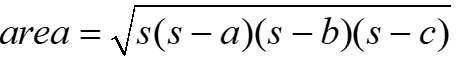• 输入：a b c三角形的三条边,可以是小数；

• 输出：三角形面积，保留3位小数。

#include<stdio.h>
int main(void)
{
/*********Begin*********/
float a,b,c,area,s;
scanf("%f %f %f",&a,&b,&c);
s=(a+b+c)/2.0;
area=sqrt(s*(s-a)*(s-b)*(s-c));
printf("%.3f",area);

/*********End**********/
return 0;
}


### 第7关立体几何计算题

• 输入：两个double型浮点数，rh

• 输出：圆周长C1、圆球表面积Sb、圆柱体积Vb。 保留两位小数，每个结果后换行。

#include<stdio.h>
#define PI 3.14
int main(void)
{
/*********Begin*********/
double r,h,C1,Sb,Vb;
scanf("%lf,%lf",&r,&h);
C1=2*PI*r;
Sb=4*PI*r*r;
Vb=PI*r*r*h;
printf("C1=%.2lf\n",C1);
printf("Sb=%.2lf\n",Sb);
printf("Vb=%.2lf\n",Vb);

/*********End**********/
return 0;
}


### 第8关计算两个正整数的最大公约数

• 输入：输入格式:"%d,%d"
• 输出：输出格式："%d\n"
#include<stdio.h>
int MaxCommonFactor( int a, int b)
{
int c;
if(a<=0||b<=0)
return -1;
while(b!=0)
{
c=a%b;
a=b;
b=c;
}
return a;
}
int main(void)
{
/*********Begin*********/
int n,m;
scanf("%d,%d",&n,&m);
printf("%d\n",MaxCommonFactor(n,m));

/*********End**********/
return 0;
}


### 第1关排序

printf("请输入三个整数:"); scanf("%d%d%d",&a,&b,&c);

printf("从小到大排列为:%d,%d,%d",a,b,c);

#include<stdio.h>
#include<math.h>
int main(void)
{
/*********Begin*********/
int a;
printf("请输入三个整数:");
for(int i=1;i<=3;i++){
scanf("%d",&a[i]);
}
for(int i=1;i<3;i++){
for(int j=i+1;j<4;j++){
if(a[i]>a[j]){
int t=a[i];
a[i]=a[j];
a[j]=t;
}
}
}
printf("从小到大排列为:%d,%d,%d",a,a,a);
/*********End**********/
return 0;
}


### 第2关选择结构-闰年判断

2000

2000 是闰年！

#include<stdio.h>
int main(void)
{
/*********Begin*********/
int year;
scanf("%d",&year);
if((year%400==0) || (year%4==0&&year%100!=0)){
printf("%d 是闰年！",year);
}
else{
printf("%d 不是闰年！",year);
}

/*********End**********/
return 0;
}


### 第3关选择结构-分段函数问题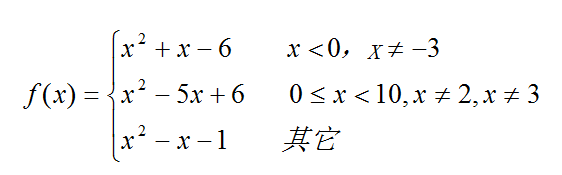#include<stdio.h>
int main(void)
{
/*********Begin*********/
double x,y;
scanf("%lf",&x);
if(x<0 && x!=-3){
y=x*x+x-6;
}
else if(x>=0 && x<10 && x!=2 && x!=3){
y=x*x-5*x+6;
}
else{
y=x*x-x-1;
}
printf("%.3f",y);

/*********End**********/
return 0;
}


### 第4关学生成绩等级换算

(1)如果输入数据在0100范围内：一个字符，表示成绩等级。 (2)如果输入数据不在0~100范围内，请输出一行：“Score is error!

#include<stdio.h>
int main(void)
{
/*********Begin*********/
int n;
scanf("%d",&n);
if(n>100 || n<0)
{
printf("Score is error!");
return 0;
}
else if(n>=90 && n<=100)
{
printf("A");
return 0;
}
else if(n>=80 && n<=89)
{
printf("B");
return 0;
}
else if(n>=70 && n<=79)
{
printf("C");
return 0;
}
else if(n>=60 && n<=69)
{
printf("D");
return 0;
}
else if(n<60)
{
printf("E");
return 0;
}

/*********End**********/
return 0;
}


### 第1关快递费用计算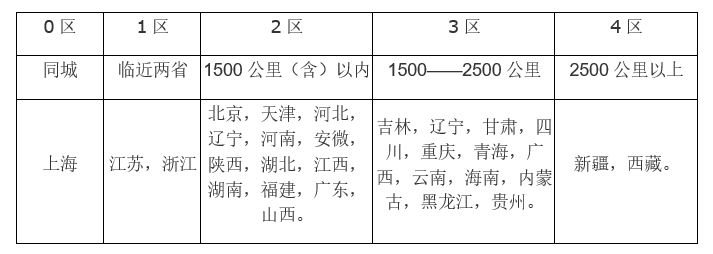（1） 起重（首重）1公斤按起重资费计算（不足1公斤，按1公斤计算），超过首重的重量，按公斤（不足1公斤，按1公斤计算）收取续重费； （2） 同城起重资费10元，续重3元/公斤； （3） 寄往1区（江浙两省）的邮件，起重资费10元，续重4元； （4） 寄往其他地区的邮件，起重资费统一为15元。而续重部分，不同区域价格不同：2区的续重5元/公斤，3区的续重6.5元/公斤，4区的续重10元/公斤。

#include<stdio.h>
int main(void)
{
int area;
float weight,price,x1,x2;
scanf("%d,%f",&area,&weight);
if (weight<=1)
{
x1=1.0;
}
else{
x1=1.0;
x2=ceil(weight-1);
}
if (area>0&&area<=4){
switch(area){
case(0):price=x1*10+x2*3;break;
case(1):price=x1*10+x2*4;break;
case(2):price=x1*15+x2*5;break;
case(3):price=x1*15+x2*6.5;break;
case(4):price=x1*15+x2*10;break;
}
printf("Price: %5.2f\n",price);
}
else {
printf("Error in Area\n");
printf("Price: 0.00\n");
}

/*********End**********/
return 0;
}


### 第2关计算一元二次方程的根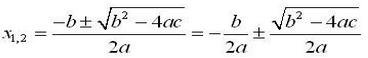#include<stdio.h>
int main(void)
{
/*********Begin*********/
float a,b,c,x1,x2;
scanf("%0f,%0f,%0f",&a,&b,&c);
x1=(-b+sqrt(b*b-4*a*c))/(2*a);
x2=(-b-sqrt(b*b-4*a*c))/(2*a);
if((b*b-4*a*c)<0)
{
printf("error!\n");
}
else
{
printf("x1=%.4f, x2=%.4f\n",x1,x2);
}

/*********End**********/
return 0;
}


### 第3关产品信息格式化

Enter item number:

385↙

Enter unit price:

12.5↙

Enter purchase date (mm/dd/yy):

12/03/2015↙

Item Unit Purchase

385 $12.50 12032015 输入 产品编号输入格式："%d" 产品价格输入格式："%f" 购买日期输入格式："%d/%d/%d" 输出 产品编号输入提示信息："Enter item number:\n" 产品价格输入提示信息："Enter unit price:\n" 购买日期输入提示信息："Enter purchase date (mm/dd/yy):\n" 格式化输出的表头信息："Item Unit Purchase\n" 输出格式："%-9d$ %-9.2f%02d%02d%02d\n"

#include<stdio.h>
int main(void)
{
/*********Begin*********/
printf("Enter item number:\n");
int n;
scanf("%d",&n);
printf("Enter unit price:\n");
float p;
scanf("%f",&p);
printf("Enter purchase date (mm/dd/yy):\n");
int m,d,y;
scanf("%d/%d/%d",&m,&d,&y);
printf("Item Unit Purchase\n");
printf("%-9d\$ %-9.2f%02d%02d%02d\n",n,p,m,d,y);

/*********End**********/
return 0;
}


### 第1关小球自由落体运动

#include<stdio.h>
int main(void)
{
/*********Begin*********/
float m,n,h,i,t,sum;
scanf("%f %f",&m,&n);
sum=m;
for(i=1;i<n;i++)
{
t=m/2;
h=t/2;
sum+=2*t;
m=t;
}
printf("%.2f %.2f",h,sum);
/*********End**********/
return 0;
}


### 第2关求解出n以内所有能被5整除的正整数的乘积

scanf("%d", &n)

printf("%d\n", s);

#include<stdio.h>
int main(void)
{
/*********Begin*********/
int n,y=1,i;
scanf("%d",&n);
for(i=5;i<=n;i+=5)y*=i;
printf("%d\n",y);
return 0;

/*********End**********/
return 0;
}


### 第3关最大公约数和最小公倍数

#include<stdio.h>
int main(void)
{
/*********Begin*********/
int x, y, z, m, n;
scanf("%d%d", &x, &y);
m = x, n = y;
while (y != 0)
{
z = x%y;
x = y;
y = z;
}
printf("最大公约数是:%d\n", x);
printf("最小公倍数是:%d", m*n / x);
/*********End**********/
return 0;
}


### 第4关字符串中各类字符数的统计

#include<stdio.h>
int main(void)
{
/*********Begin*********/

int letter=0,space=0,digit=0,other=0;/*定义变量并初始化*/
char c;/*定义字符串c*/
while((c=getchar())!='\n')/*判断c是否是回车键*/
if(c>='a'&&c<='z'||c>='A'&&c<='Z')/*判断c是否是字母*/
letter++;/*计算字母个数*/
else if(c==' ')/*判断c是否是空格*/
space++;/*计算空格个数*/
else if(c>='0'&&c<='9')/*判断c是否是数字*/
digit++;/*计算数字个数*/
else
other++;/*计算其他字符个数*/
printf("%d %d %d %d",letter,digit,space,other);

/*********End**********/
return 0;
}


### 第5关求sn=a+aa+aaa+aaaa+......的值

C中可利用whilefordo-while等常用循环函数来是语句或代码块进行重复操作，从而省去很多重复的工作，使代码简洁明了。

while语句

1. while(布尔值){
2. 需要循环的语句或者代码块
3. }
• 其中括号中的布尔值如果为真，这进入循环，直到布尔值变为假，则推出循环，继续执行之后代码。
• 其中括号中的布尔值如果为假，则直接不进入循环，继续执行循环代码块之后代码。

1. // 需要循环输出3次6
2. int n = 3;
3. while(n--){
4. printf("6");
5. }

for语句

1. for(1.赋值语句或者省略;2.判断语句或者省略；3.执行语句或者省略){
2. 需循环的语句或者代码块
3. }
• 其中1处位置，是一个赋值语句，在循环开始前执行一次，之后再不执行。
• 其中2处位置为判断语句，每次开始循环时（包括第一次）都会先经过这个判断语句的判断
• 如果此处为真，则进入循环，执行循环内语句或者代码块。
• 如果此处为假，则不进入循环，执行之后的代码。
• 其中3处位置为一个运算语句，每执行完一次循环，就会执行一次此处代码。

1. // 同样需要输出3个6
2. int i;
3. for(i=0;i<3;i++){
4. printf("6");
5. }

#include<stdio.h>
int main(void)
{
/*********Begin*********/
int a,n,i;
double sum;
scanf("%d %d",&a,&n);
for(i=n-1,sum=0;i>=0;i--)
sum+=(pow(a,(n-i)));

for(i=n-1,sum=n*a;i>0;i--)
sum+=(n-i)*a*(pow(10,i));

printf("%.0lf",sum);

/*********End**********/
return 0;
}


### 第1关C循环-求平均成绩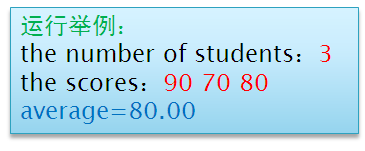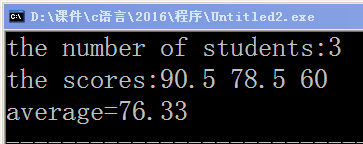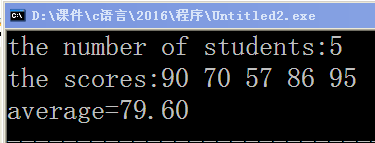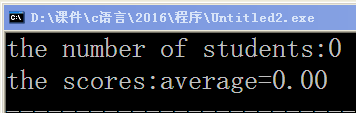C中可利用whilefordo-while等常用循环函数来是语句或代码块进行重复操作，从而省去很多重复的工作，使代码简洁明了。

while语句

1. while(布尔值){
2. 需要循环的语句或者代码块
3. }
• 其中括号中的布尔值如果为真，这进入循环，直到布尔值变为假，则推出循环，继续执行之后代码。
• 其中括号中的布尔值如果为假，则直接不进入循环，继续执行循环代码块之后代码。

1. // 需要循环输出3次6
2. int n = 3;
3. while(n--){
4. printf("6");
5. }

for语句

1. for(1.赋值语句或者省略;2.判断语句或者省略；3.执行语句或者省略){
2. 需循环的语句或者代码块
3. }
• 其中1处位置，是一个赋值语句，在循环开始前执行一次，之后再不执行。
• 其中2处位置为判断语句，每次开始循环时（包括第一次）都会先经过这个判断语句的判断
• 如果此处为真，则进入循环，执行循环内语句或者代码块。
• 如果此处为假，则不进入循环，执行之后的代码。
• 其中3处位置为一个运算语句，每执行完一次循环，就会执行一次此处代码。

1. // 同样需要输出3个6
2. int i;
3. for(i=0;i<3;i++){
4. printf("6");
5. }-

1. #include <stdio.h>
2. // 定义main函数
3. int main()
4. {
5. // 请在此添加代码
6. /********** Begin *********/
7. /********** End **********/
8. return 0;
9. }
#include<stdio.h>
int main(void)
{
/*********Begin*********/
int n,i;
float score,sum=0,average;
scanf("%d",&n);
for(i=1;i<=n;i++)
{
scanf("%f",&score);
sum+=score;
}
average=(n<=0?0:sum/n);
printf("the number of students:the scores:average=%.2f",average);

/*********End**********/
return 0;
}


### 第2关C循环-求各位数字之积

C中可利用whilefordo-while等常用循环函数来是语句或代码块进行重复操作，从而省去很多重复的工作，使代码简洁明了。

while语句

1. while(布尔值){
2. 需要循环的语句或者代码块
3. }
• 其中括号中的布尔值如果为真，这进入循环，直到布尔值变为假，则推出循环，继续执行之后代码。
• 其中括号中的布尔值如果为假，则直接不进入循环，继续执行循环代码块之后代码。

1. // 需要循环输出3次6
2. int n = 3;
3. while(n--){
4. printf("6");
5. }

for语句

1. for(1.赋值语句或者省略;2.判断语句或者省略；3.执行语句或者省略){
2. 需循环的语句或者代码块
3. }
• 其中1处位置，是一个赋值语句，在循环开始前执行一次，之后再不执行。
• 其中2处位置为判断语句，每次开始循环时（包括第一次）都会先经过这个判断语句的判断
• 如果此处为真，则进入循环，执行循环内语句或者代码块。
• 如果此处为假，则不进入循环，执行之后的代码。
• 其中3处位置为一个运算语句，每执行完一次循环，就会执行一次此处代码。

1. // 同样需要输出3个6
2. int i;
3. for(i=0;i<3;i++){
4. printf("6");
5. }

1. #include <stdio.h>
2. // 定义main函数
3. int main()
4. {
5. // 请在此添加代码
6. /********** Begin *********/
7. /********** End **********/
8. return 0;
9. }
#include<stdio.h>
int main(void)
{
/*********Begin*********/
int fac=1,num;
scanf("%d",&num);
while(num)
{
fac*=num%10;
num/=10;
}
printf("%d",fac);

/*********End**********/
return 0;
}


### 第3关C循环-求阶乘之和

C中可利用whilefordo-while等常用循环函数来是语句或代码块进行重复操作，从而省去很多重复的工作，使代码简洁明了。

while语句

1. while(布尔值){
2. 需要循环的语句或者代码块
3. }
• 其中括号中的布尔值如果为真，这进入循环，直到布尔值变为假，则推出循环，继续执行之后代码。
• 其中括号中的布尔值如果为假，则直接不进入循环，继续执行循环代码块之后代码。

1. // 需要循环输出3次6
2. int n = 3;
3. while(n--){
4. printf("6");
5. }

for语句

1. for(1.赋值语句或者省略;2.判断语句或者省略；3.执行语句或者省略){
2. 需循环的语句或者代码块
3. }
• 其中1处位置，是一个赋值语句，在循环开始前执行一次，之后再不执行。
• 其中2处位置为判断语句，每次开始循环时（包括第一次）都会先经过这个判断语句的判断
• 如果此处为真，则进入循环，执行循环内语句或者代码块。
• 如果此处为假，则不进入循环，执行之后的代码。
• 其中3处位置为一个运算语句，每执行完一次循环，就会执行一次此处代码。

1. // 同样需要输出3个6
2. int i;
3. for(i=0;i<3;i++){
4. printf("6");
5. }

1. #include <stdio.h>
2. // 定义main函数
3. int main()
4. {
5. // 请在此添加代码
6. /********** Begin *********/
7. /********** End **********/
8. return 0;
9. }
#include<stdio.h>
int main(void)
{
/*********Begin*********/
int i, n, sum = 0, f = 1;
scanf("%d", &n);
for(i = 1; i <= n; i++)
{
f*=i;
sum+=f;
}
printf("%ld",sum);

/*********End**********/
return 0;
}


### 第4关C循环-水仙花数

C中可利用whilefordo-while等常用循环函数来是语句或代码块进行重复操作，从而省去很多重复的工作，使代码简洁明了。

while语句

1. while(布尔值){
2. 需要循环的语句或者代码块
3. }
• 其中括号中的布尔值如果为真，这进入循环，直到布尔值变为假，则推出循环，继续执行之后代码。
• 其中括号中的布尔值如果为假，则直接不进入循环，继续执行循环代码块之后代码。

1. // 需要循环输出3次6
2. int n = 3;
3. while(n--){
4. printf("6");
5. }

for语句

1. for(1.赋值语句或者省略;2.判断语句或者省略；3.执行语句或者省略){
2. 需循环的语句或者代码块
3. }
• 其中1处位置，是一个赋值语句，在循环开始前执行一次，之后再不执行。
• 其中2处位置为判断语句，每次开始循环时（包括第一次）都会先经过这个判断语句的判断
• 如果此处为真，则进入循环，执行循环内语句或者代码块。
• 如果此处为假，则不进入循环，执行之后的代码。
• 其中3处位置为一个运算语句，每执行完一次循环，就会执行一次此处代码。

1. // 同样需要输出3个6
2. int i;
3. for(i=0;i<3;i++){
4. printf("6");
5. }

· 注意：本题不需要输入语句，由于网站限制要求一定要有输入输出示例，但同学们可以对输入部分忽略不计。 例如： 370就是一个水仙花数，因为370 = 3^3 +7^3 + 0^3

#include<stdio.h>
int main(void)
{
/*********Begin*********/
int hun, ten, ind,a, n;
a=getchar();
while(a!=1)
break;
for( n=100; n<1000; n++ )  /*整数的取值范围*/
{
hun = n / 100;
ten = (n-hun*100) / 10;
ind = n % 10;
if(n == hun*hun*hun + ten*ten*ten + ind*ind*ind)  /*各位上的立方和是否与原数n相等*/
printf("%d ", n);
}
/*********End**********/
return 0;
}


### 第5关C循环-寻找完数

1000

#include <stdio.h>
int is_perfect_number(int n)
{
int i,s=0;
for(i = 1; i <=n/2; i ++)
if(n%i == 0) s+= i;//统计所有真因子的和。
if(s == n) return 1;//如果与原值相等，则该数为完数。
return 0;//不是完数。
}
int main()
{
int n,x;
x=getchar();
for(n = 1; n < 1000; n++)
if(is_perfect_number(n))
printf("%d\n",n);
}



### 第6关分数求和

#include <stdio.h>
#include <math.h>
int main()
{
int i = 0;
double sum = 0.0;
for (i = 1; i <= 100; i++)
{
sum = sum + (pow(-1, i + 1)) / i;
}
printf("%.3f", sum);
return 0;
}



### 第1关求和

#include<stdio.h>
//编写函数
/*********Begin*********/
int f(int n)
{
int s=0;
for(int i=1;i<=n;i++)
{
s+=i;
}
return s;
}
/*********End**********/
int main(void)
{
/*********Begin*********/
int n;
scanf("%d",&n);
int y=f(n);
printf("%d",y);

/*********End**********/
return 0;
}


### 第2关回文数计算

202 212 222 232 242 .....

#include<stdio.h>
void solve(){
/*********Begin*********/

int a,b,c,m,n,p,q;

for(int i = 200 ; i <= 3000 ; i++ ) {
if( i < 1000 ) {
c = i%10;
b = (i/10)%10;
a = i/100;
if( i == c*100 + b*10 + c ) {
printf("%d\n", i);
}
} else {
m = i/1000;
n = (i/100)%10;
p = (i/10)%10;
q = i%10;
if( i == q*1000 + p*100 + n*10 + m ) {
printf("%d\n", i);
}
}

}
return 0;

/*********End**********/
}
int main(void)
{
solve();
return 0;
}



### 第3关 编写函数求表达式的值

#include<stdio.h>
//编写题目要求的函数
/*********Begin*********/

/*********End**********/
int main()
{
/*********Begin*********/

double ans = 1;
int n;
scanf("%d", &n);
double pre_1 = 1;
double pre_2 = 1;
for(int i = 1; i <= n; i ++)
{
pre_1 *= i;
pre_2 *= (2 * i + 1);
ans += pre_1 * 1.0 / pre_2;
}
printf("%.10f", ans);
return 0;

/*********End**********/

}


### 第4关阶乘数列

#include<stdio.h>
#define ll long long
ll a;

void csh()
{
a = 1;
for(ll i = 1; i <= 20; i ++)
{
a[i] = a[i - 1] * i;
}
}

int main()
{
csh();
int n;
scanf("%d", &n);
ll sum = 0;
for(int i = 1; i <= n; i ++)
{
sum += a[i];
}
printf("%lld", sum);
return 0;
}


### 第5关亲密数

3000以内的全部亲密数(输出格式：(A,B)，不加换行，不加分隔符号) 一对亲密数只输出一次，小的在前。

#include<stdio.h>

int main(void)
{
printf("(220,284)(1184,1210)(2620,2924)");
return 0;
}


### 第6关公约公倍数

#include<stdio.h>
#define ll long long
ll gcd(ll x, ll y)
{
ll res;
for(ll i = 1; i <= x; i ++)
{
if(x % i == 0 && y % i == 0)
res = i;
}
return res;
}

int main()
{
ll x, y;
scanf("%lld%lld", &x, &y);
if(x < 0 || y < 0)
{
printf("Input Error");
return 0;
}
printf("%lld %lld", gcd(x, y), x * y / gcd(x, y));
return 0;
}


### 第1关递归求阶乘数列

5

153

#include<stdio.h>
long long solve(long long n){
/*********Begin*********/
long long m=1,s=0;
for(int i=1;i<=n;i++){
m*=i;
s+=m;
}
return s;
/*********End**********/
}
int main(void)
{
long long n;
scanf("%lld",&n);
long long ans=0;

ans=solve(n);
printf("%lld", ans);
return 0;
}


### 第2关递归实现输出一个整数的逆序

#include<stdio.h>
void solve(int n){
printf("%d", n%10);
/*********Begin*********/
n/=10;
/*********End**********/
if(n>0) solve(n);
}
int main(void)
{
int n;
scanf("%d",&n);
solve(n);
return 0;
}


### 第3关将整数递归转换成字符串

#include<stdio.h>
void solve(int n){
int temp=n%10;
/*********Begin*********/
n/=10;
if(n!=0)solve(n);

/*********End**********/
if(n)
printf(" %d", temp);
else
printf("%d", temp);
}
int main(void)
{
int n;
scanf("%d",&n);
solve(n);
return 0;
}


### 第4关递归实现Ackman函数

Acm(m,n)= ⎩⎪⎪⎨⎪⎪⎧​n+1Acm(m−1,1)Acm(m−1,Acm(m,n−1))​ if m=0,n>0 if n=0,m>0 if n>0,m>0​

#include<stdio.h>
int Acm(int m,int n){
if(m==0&&n>0)
/*********Begin*********/
return (n+1);
/*********End**********/
else if(n==0&&m>0)
/*********Begin*********/
return Acm(m-1,1);
/*********End**********/
else
/*********Begin*********/
return Acm(m-1,Acm(m,n-1));
/*********End**********/
}
int main(void)
{
int m,n;
scanf("%d%d",&m,&n);
printf("%d", Acm(m,n));
return 0;
}


### 第1关排序问题

#include<stdio.h>
int main(void)
{
/*********Begin*********/
int i,j,a,t;
for (i = 0; i < 10; i++)
scanf("%d",&a[i]);
for (i = 0; i < 10; i++)
{
for (j = i + 1; j < 10;j++)
if (a[i] < a[j])
{
t = a[i];
a[i] = a[j];
a[j] = t;
}
}
for (i = 0; i < 10; i++)
{
printf("%d", a[i]);
if(i!=9)
putchar(' ');}
/*********End**********/
return 0;
}

### 第2关查找整数

#include<stdio.h>
int Find(int n,int a[],int key)
{
int index=-1;
int i;
for(i=0;i<n;i++)
{
if(a[i]==key)
{
index=i+1;
break;
}
}
return index;
}
int main()
{
int n,a;
int i;
int aa;
scanf("%d",&n);
for(i=0;i<n;i++)
{
scanf("%d",&aa[i]);
}
scanf("%d",&a);
printf("%d",Find(n,aa,a));
return 0;
}

### 第3关计算数组中元素的最大值及其所在的行列下标值

5,5 1 2 3 4 5 4 5 6 100 2 3 2 1 5 6 1 2 3 5 4 3 5 6 4 8

Input m, n:Input 5*5 array: max=100, row=2, col=4

#include<stdio.h>
int main(void)
{
/*********Begin*********/
int a,max,i,j,p,q,m,n;
printf("Input m, n:");
scanf("%d,%d",&m,&n);
printf("Input %d*%d array:\n",m,n);
for(i=0;i<m;i++)
for(j=0;j<n;j++)
scanf("%d",&a[i][j]);
max=a;
p=q=0;
for(i=0;i<m;i++)
for(j=0;j<n;j++)
if(a[i][j]>max){
max=a[i][j];
p=i;
q=j;
}
printf("max=%d, row=%d, col=%d",max,p+1,q+1);
/*********End**********/
return 0;
}

### 第4关二分查找

#include <stdio.h>
int a,n,t;
int BS(){
int l=0,r=n-1;
while(l<=r){
int m=(l+r)>>1;
if(t<a[m])
r=m-1;
else if(t>a[m])
l=m+1;
else if(a[m-1]<t)
return m;
else r=m-1;
}
return -1;
}
int main(){
while(~scanf("%d",&n)){
for(int i=0;i<n;i++)
scanf("%d",&a[i]);
scanf("%d",&t);
if(a==t)
printf("1");
else{
int f=BS();
if(f!=-1){
printf("%d",f+1);
}
else
printf("None");
}}
return 0;
}


### 第5关鞍点

#define N 10
#include <stdio.h>
int Maxcol(int a[][N],int n,int row){
int i,maxcol=0;
for(i=1;i<n;i++)
if (a[row][i]>a[row][maxcol]) maxcol=i;
return maxcol;
}
int Minrow(int a[][N],int m,int col){
int i,minrow=0;
for(i=1;i<m;i++)
if (a[i][col]<a[minrow][col]) minrow=i;
return minrow;
}
int main(){
int m,n,i,j;
int maxcol,minrow;
int a[N][N];
scanf("%d%d",&m,&n);
for(i=0;i<m;i++) for(j=0;j<n;j++)
scanf("%d",&a[i][j]);
for(i=0;i<m;i++){
maxcol=Maxcol(a,n,i);
minrow=Minrow(a,m,maxcol);
if (i==minrow){
printf("Array[%d][%d]=%d",i,maxcol,a[i][maxcol]);
break;
}
}
if(i>=m) printf("None");
}

### 第6关删除最大值

#include<stdio.h>
int main()
{
int a;
int MAX = -1;
for(int i = 0; i < 10; i ++)
scanf("%d", &a[i]);
for(int i = 0; i < 10; i ++)
{
if(a[i] > MAX) MAX = a[i];
}
for(int i = 0; i < 10; i ++)
{
if(a[i] == MAX) continue;
printf("%d ", a[i]);
}
return 0;
}



### 第7关杨辉三角

#include<stdio.h>
int a;

int main()
{
int num = 1;
for(int i = 0; i < 10; i ++) a[i] = 1;
for(int i = 1; i < 10; i ++)
{
for(int j = 1; j < 10; j ++)
{
if(j == num)
{
num ++;
a[i][j] = 1;
break;
}
a[i][j] = a[i - 1][j - 1] + a[i - 1][j];
}
}
num = 1;
for(int i = 0; i < 10; i ++)
{
for(int j = 0; j < 10; j ++)
{
if(j == num)
{
num ++;
break;
}
if(j == num - 1) printf("%d", a[i][j]);
else printf("%d ", a[i][j]);
}
printf("\n");
}
return 0;
}

### 第1关字符逆序

#include<stdio.h>
int main(void)
{
/*********Begin*********/
char n;
int i=0,temp;
int len=0;
gets(n);
while(n[i]!=0)
{
len++;
i++;
}
int left=0,right=len-1;
while(left<right)
{
temp=n[left];
n[left]=n[right];
n[right]=temp;
left++;
right--;
}
for(i=0;i<len;i++)
printf("%c",n[i]);
/*********End**********/
return 0;
}

### 第2关字符统计

#include<stdio.h>
int main(void)
{
/*********Begin*********/
int n,count,i,k,j,w;
char a;
scanf("%d",&n);
w = n;
int b={0};

while(n > 0)
{
count = 0;
scanf("%s",a);
k = strlen(a);

for(i=0; i<k; i++)
{
if(a[i]<='9' && a[i]>='0')
{
count++;
}
}
b[n-1] = count;
n--;
}
for(j=w-1; j>=0; j--)
{
printf("%d", b[j]);
if(j>0)
printf("\n");
}

/*********End**********/
return 0;
}

### 第3关字符插入

#include<stdio.h>
#include<string.h>
int main()
{
char a, b;
int i = 0, j = 0, min, max, x, y;
min = 0;
max = 0;
scanf("%s",a);
scanf("%s",b);
x = strlen(a);
y = strlen(b);
for (i = 0; i<x; i++)
{
if (a[i]<a[min])
min = i;
}
for (j = 0; j<y; j++)
{
if (b[j]>b[max])
max = j;
}

for (i = x; i >min; i--)
{
a[i] = a[i-1];
}
if (min == x)
a[i] = b[max];
else
{
a[i +1] = b[max];
}
a[x + 1] = '\0';
printf("%s",a);
}

### 第4关字符串处理

#include<stdio.h>
int main(void)
{
/*********Begin*********/
char t,s;
int i,j,pos;
gets(t);
gets(s);
scanf("%d",&pos);
for(i=0;i<strlen(t);i++)
{
printf("%c",t[i]);
if(i+1==pos)
{
for(j=0;j<strlen(s);j++)
printf("%c",s[j]);
}
}
/*********End**********/
return 0;
}

### 第5关字符串统计

#include<stdio.h>
#include <string.h>
int main(void)
{
/*********Begin*********/
char a;
int i, j, pos = 0;
int str_len, word_len, max_word_len;

while(1) {
str_len = word_len = max_word_len = 0;

fgets(a, 100, stdin);//fgets函数的用法

if (strlen(a) <= 1)//输入的字符只有一个的情况
continue;
if (strlen(a) < 99)   //remove '\n'
a[strlen(a)-1] = 0;

if(strncmp(a,"stop", strlen("stop"))==0)
break;
for(i = 0; a[i] !='\0'; i++) {
if(a[i] != ' ') {
word_len++;
str_len++;
continue;
}
if (word_len  > max_word_len) {
max_word_len = word_len;
pos = i - word_len;
}
word_len = 0;
}
if (word_len  > max_word_len) {
max_word_len = word_len;
pos = i - word_len;
}

printf("%d ", str_len);
for (i = pos; i < pos + max_word_len; i++)
printf("%c", a[i]);
putchar(10);
}

/*********End**********/
return 0;
}

### 第6关字符串排序

#include<stdio.h>
#include<string.h>
int main(void)
{
/*********Begin*********/
char a,b,c,max;
scanf("%s %s %s",&a,&b,&c);
if(strcmp(a,b)>0)
{
strcpy(max,a);
strcpy(a,b);
strcpy(b,max);
}
if(strcmp(a,c)>0)
{
strcpy(max,a);
strcpy(a,c);
strcpy(c,max);
}
if(strcmp(b,c)>0)
{
strcpy(max,b);
strcpy(b,c);
strcpy(c,max);
}
printf("%s\n%s\n%s",a,b,c);
/*********End**********/
return 0;
}

### 第1关用指针法输入12个整数,然后按每行4个数输出

1 2 3 4 5 6 7 8 9 10 11 12

1 2 3 4 5 6 7 8 9 10 11 12

#include<stdio.h>
int main(void)
{
int a,n,i;
int *b=a;
for(n=0;n<12;n++){
scanf("%d",b++);
}
b=a;
i=1;
for(n=0;n<12;n++){
if((n+1)/4==i){
printf("%d",*b);
}
else {
printf("%d ", *b);
}
b++;
if((n+1)/4==i){
if (i==3){
break;
}
printf("\n");
i++;
}
}
return 0;
}

### 第2关指针变量作为函数参数实现两变量交换值

输出用函数交换处理后的值a b

#include "stdio.h"
void swap2(int *p1,int *p2)
{
int temp;
temp = *p1;
*p1 = *p2;
*p2 = temp;
}
int main()
{

//        int c = 3,d = 4;
//        printf("swap2交换c-d之前：%d,%d\n",c,d);
int c,d;
scanf("%d %d",&c,&d);

swap2(&c,&d);
//    printf("swap2交换c-d之后：%d,%d\n",c,d);
printf("%d %d",c,d);
}


### 第3关报数

#include "stdio.h"
int main(int argc,char *argv[])
{
int n,ans,i;
scanf("%d",&n);
ans=0;
if(n==0)
return 0;
for(i=2;i<=n;i++)
ans=(ans+3)%i;
printf("%d\n",ans%n+1);
return 0;
}

### 第4关strcmp函数

abc abd

ab

#include<stdio.h>
int strcmp(char *p1,char *p2)
{
/*********Begin*********/
int i;

i=0;
while(*(p1+i)==*(p2+2))
{
if(*(p1+i++)=='\0')
{
return 0;
}
}
return (*(p1+i)-(*(p2+i)));
/*********End**********/
}
int main(void)
{
char a,b;
scanf("%s%s",a,b);
if(strcmp(a,b)>0)
printf("%s", a);
else
printf("%s", b);

return 0;
}

### 第1关输出若干个学生成绩中的最高分.要求用指针函数实现

5 80 90 85 95 91

95

#include <stdio.h>
int max(int *a,int *b);
int main()
{
int sum,n,c;
scanf("%d",&sum);
int q[sum];
for(n=0;n<sum;n++)
{
scanf("%d",&q[n]);
}
for(n=0;n<(sum-1);n++)
{
c=max(&q[n],&q[n+1]);
}
printf("%d",c);

}
int max(int *a,int *b)
{
int r;
r=*a>*b?*a:*b;
return(r);
}

### 第2关采用指针变量表示地址的方法输入输出数组中的个元素

10 1 2 3 4 5 6 7 8 9 10

1 2 3 4 5 6 7 8 9 10

#include<stdio.h>
int main(void)
{
/*********Begin*********/
int n,i;int a;int *p=a;
scanf("%d",&n);
for(i=0;i<n;i++,p++){
scanf("%d",p);
}
for(p=a;p<a+n;p++){
printf("%d ",*p);
}

/*********End**********/
return 0;
}

### 第3关用指针实现数组循环移动

10 5 1 2 3 4 5 6 7 8 9 0

6 7 8 9 0 1 2 3 4 5

#include<stdio.h>
int move(int *a,int n,int m)
{
int t;
int i;
for(i=0;i<n;i++)
{
t[i]=a[i];
}
for(i=0;i<m;i++)
{
a[i]=t[n-m+i];
}
for(i=m;i<n;i++)
{
a[i]=t[i-m];
}
}
int main()
{
int a;
int n,m;
int i;
while(scanf("%d%d",&n,&m)!=EOF)
{
for(i=0;i<n;i++)
{
scanf("%d",&a[i]);
}
move(a,n,m);
for(i=0;i<n-1;i++)
{
printf("%d ",a[i]);
}
printf("%d\n",a[i]);
}
}


### 第1关结构体变量的初始化和引用

1. struct <结构体类型名>
2. {
3. <成员表>
4. }; //分号结束定义

1. struct student{
2. long number;
3. char name;
4. char sex;
5. float score;
6. };

• 先定义结构体，再声明结构体变量

1. struct student{
2. long number;
3. char name;
4. char sex;
5. float score;
6. }; //先定义结构体
7. struct student s1,s2;//声明结构体变量
• 在定义结构体类型的同时声明结题变量

1. struct student{
2. long number;
3. char name;
4. char sex;
5. float score;
6. }s1,s2; //在定义结构体的同时定义结构体变量
• 直接声明结构体变量

1. struct{ //省去结构体名
2. long number;
3. char name;
4. char sex;
5. float score;
6. }s1,s2; //直接给出结构体变量

• typedef引用别名来定义

1. typedef struct student STUDENT; //给结构体student定义别名
2. struct student{
3. long number;
4. char name;
5. char sex;
6. float score;
7. };
8. STUDENT s1,s2; //使用别名STUDENT定义结构体

1. s1.number=2010001;//number成员是long类型
2. strcpy(s1.name,"lili")；//name成员是字符数组类型

1. struct data{
2. int month;
3. int day;
4. int year;
5. };
6. struct student{
7. long num;
8. char name;
9. char sex;
10. data birthday;
11. }stu1;

1. stu1.num;
2. stu1.birthday.month;

#include<stdio.h>
struct Student{
long number;
char name;
int score;
};
int main(){
struct Student arr;
struct Student *p;
p=arr;
scanf("%ld %s %d",&(*p).number,(*p).name,&(*p).score);
p++;
scanf("%ld %s %d",&(*p).number,(*p).name,&(*p).score);
p=arr;
if(p->score >(p+1)->score){
printf("%ld %s %d",(*p).number,(*p).name,(*p).score);
}else{
p++;
printf("%ld %s %d",(*p).number,(*p).name,(*p).score);
}
return 0;
}



### 第2关结构体排序

#include<stdio.h>
/*********Begin*********/
struct Student{
long number;
char name;
int score;
};

/*********End**********/
int main(void)
{
/*********Begin*********/
int n,i,k,j;
scanf("%d",&n);
struct Student arr;
struct Student t;
struct Student *p=arr;
for(i=0;i<n;i++,p++){
scanf("%ld %s %d",&p->number,p->name,&p->score);
}

for(i=0;i<n-1;i++){
k=i;
for(j=i+1;j<n;j++){
if(arr[k].score<arr[j].score){
k=j;
}
}
t=arr[k];arr[k]=arr[i];arr[i]=t;
}
p=arr;
for(i=0;i<n;i++,p++){
printf("%ld %s %d",p->number,p->name,p->score);
printf("\n");
}

/*********End**********/
return 0;
}

### 第3关结构体存储数据

#include <stdio.h>
#include <string.h>
struct  person
{
char name;
int count;
};
int main()
{
int i,j,k;
char name;
scanf("%d",&k);
for(i=1;i<=k;i++)
{
/***** 在以下一行填写代码 *****/
//输入候选人姓名
char n ;
scanf("%s",&n);
for(j=0;j<3;j++)
/***** 在以下一行填写代码 *****/
{
{

break;
}
}
}
for(j=0;j<3;j++)
/***** 在以下一行填写代码 *****/
return 0;
}

### 第4关结构体存储学生信息

#include<stdio.h>
#include<string.h>
int Count;
struct student{
char sno,name;
int math,english,chinese,sum;
};
void print(struct student stu) {
printf("%s %s %d %d %d %d\n", stu.sno, stu.name, stu.math, stu.english, stu.chinese, stu.sum);
}
void query_stu(struct student s[],char *name);
void delete_stu(struct student s[],char *sno);
void update_stu(struct student s[],char *sno,int math,int english,int chinese);
int main(void)//涓诲嚱鏁
{
int n,q;
struct student students;
scanf("%d%d",&n,&q);
Count=n;
for(int i=0;i<n;i++){
scanf("%s %s %d %d %d",students[i].sno, students[i].name, &students[i].math, &students[i].english, &students[i].chinese);
students[n].sum=students[i].math+students[i].english+students[i].chinese;
}
while(q--){
int op;
scanf("%d",&op);
char sno,name;
if(op==1){
scanf("%s",name);
query_stu(students,name);
}
else if(op==2){
int a,b,c;
scanf("%s%d%d%d",sno,&a,&b,&c);
update_stu(students,sno,a,b,c);
for(int i=0;i<Count;i++)
print(students[i]);
}
else{
scanf("%s",sno);
delete_stu(students,sno);
for(int i=0;i<Count-1;i++)
print(students[i]);
}
}
return 0;
}
void query_stu(struct student s[],char *name) {
int n;
for (n = 0; n < Count; n++) {
if (strcmp(name, s[n].name) == 0) {
s[n].sum=s[n].chinese+s[n].english+s[n].math;
print(s[n]);
}
s[n].sum=s[n].math+s[n].english+s[n].chinese;
}
}
void update_stu(struct student s[],char *sno,int math,int english,int chinese){
int n;
for(n=0;n<Count;n++) {
if (strcmp(s[n].sno,sno)==0) {
s[n].math=math;s[n].english=english;s[n].chinese=chinese;
s[n].sum=s[n].math+s[n].english+s[n].chinese;
}
}
}
void delete_stu(struct student s[],char *sno) {
int i;
for (i = 0; i < Count - 1; i++) {
if (strlen(s[i].sno) >= strlen(sno))
if (strcmp(s[i].sno, sno) >= 0) {
s[i]=s[i+1];
}
}
}



### 第1关HelloWorld

#include<stdio.h>
void solve(){
/********** Begin *********/
FILE * pfile = fopen("in.txt","w");
fprintf(pfile,"HelloWorld");
fclose(pfile);
/********** End **********/
}

### 第2关文件读取和写入

#include<stdio.h>
void solve(){
/********** Begin *********/
int a,b,c;
FILE * r=fopen("a.txt","r");
FILE * w=fopen("b.txt","w");
while(fscanf(r,"%d%d%d",&a,&b,&c)!=EOF)
fprintf(w,"%d %d %d",a,b,c);
fclose(r);
fclose(w);

/********** End **********/
}


### 第3关统计文本字母数量

#include<stdio.h>
void solve(){
/********** Begin *********/
FILE *fp;
char word;
int num=0;
fp=fopen("a.txt","r");
while(!feof(fp))
{
fscanf(fp,"%c",&word);
if((word>='A'&&word<='Z')||(word>='a'&&word<='z'))
{
num++;
}
}
printf("%d",num-1);
fclose(fp);
/********** End **********/
}


### 第4关读取文件中指定学生信息

#include<stdio.h>
void solve(char s[]){
/********** Begin *********/
FILE *fq = fopen("a.txt", "r");
int n;
char line, a;
for (n = 0; n < 9; n++) {
fgets(line, 12, fq);
fgets(a, 100, fq);
if (strcmp(s, line) == 0) {
printf("%s%s", line, a);
//fclose(fq);
break;
}
if (n == 8) {
}
}

/********** End **********/
}


11-09
03-04
03-1620万+
06-082万+
01-048003
07-01
12-061万+
08-13
01-049925
01-23
05-24783
01-06
09-08
06-161837

### “相关推荐”对你有帮助么？

•非常没帮助
•没帮助
•一般
•有帮助
•非常有帮助被折叠的  条评论 为什么被折叠?到【灌水乐园】发言¥2 ¥4 ¥6 ¥10 ¥20余额支付 (余额：-- )扫码支付获取中扫码支付点击重新获取扫码支付1.余额是钱包充值的虚拟货币，按照1:1的比例进行支付金额的抵扣。
2.余额无法直接购买下载，可以购买VIP、C币套餐、付费专栏及课程。余额充值